# There

There is a triangle ABC: A (-2,3), B (4, -1), C (2,5). Determine the general equations of the lines on which they lie:
a) AB side,
b) height to side c,
c) Axis of the AB side,
d) median ta to side a

Result

p1 = (Correct answer is: p1)p2 = (Correct answer is: p2)p3 = (Correct answer is: p3)p4 = (Correct answer is: p4)### Step-by-step explanation: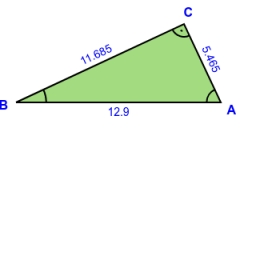Did you find an error or inaccuracy? Feel free to write us. Thank you!Tips to related online calculators
Line slope calculator is helpful for basic calculations in analytic geometry. The coordinates of two points in the plane calculate slope, normal and parametric line equation(s), slope, directional angle, direction vector, the length of the segment, intersections of the coordinate axes, etc.
Do you have a linear equation or system of equations and looking for its solution? Or do you have a quadratic equation?

#### You need to know the following knowledge to solve this word math problem:

We encourage you to watch this tutorial video on this math problem:

## Related math problems and questions:

• General line equationsIn all examples, write the GENERAL EQUATION OF a line that is given in some way. A) the line is given parametrically: x = - 4 + 2p, y = 2 - 3p B) the line is given by the slope form: y = 3x - 1 C) the line is given by two points: A [3; -3], B [-5; 2] D) t
• CoordinatesDetermine the coordinates of the vertices and the content of the parallelogram, the two sides of which lie on the lines 8x + 3y + 1 = 0, 2x + y-1 = 0 and the diagonal on the line 3x + 2y + 3 = 0
• Three parallelsThe vertices of an equilateral triangle lie on 3 different parallel lines. The middle line is 5 m and 3 m distant from the end lines. Calculate the height of this triangle.
• On lineOn line p: x = 4 + t, y = 3 + 2t, t is R, find point C, which has the same distance from points A [1,2] and B [-1,0].
• Sphere equationObtain the equation of sphere its centre on the line 3x+2z=0=4x-5y and passes through the points (0,-2,-4) and (2,-1,1).
• Three points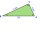Three points K (-3; 2), L (-1; 4), M (3, -4) are given. Find out: (a) whether the triangle KLM is right b) calculate the length of the line to the k side c) write the coordinates of the vector LM d) write the directional form of the KM side e) write the d
• Median or middle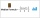The number of hours of television watched per day by a sample of 28 people is given below: 4, 1, 5, 5, 2, 5, 4, 4, 2, 3, 6, 8, 3, 5, 2, 0, 3, 5, 9, 4, 5, 2, 1, 3, 4, 7, 2, 9 What is the median value?
• A Cartesian framework1. In a Cartesian framework, the functions f and g we know that: the function (f) is defined by f (x) = 2x ^ 2, the function (g) is defined by g (x) = x + 3, the point (O) is the origin of the reference, point (C) is the point of intersection of the graph
• On a lineOn a line p : 3 x - 4 y - 3 = 0, determine the point C equidistant from points A[4, 4] and B[7, 1].
• Three points 2The three points A(3, 8), B(6, 2) and C(10, 2). The point D is such that the line DA is perpendicular to AB, and DC is parallel to AB. Calculate the coordinates of D.
• Parametric formCalculate the distance of point A [2,1] from the line p: X = -1 + 3 t Y = 5-4 t Line p has a parametric form of the line equation. ..
• CircleThe circle touches two parallel lines p and q, and its center lies on line a, which is the secant of lines p and q. Write the equation of the circle and determine the coordinates of the center and radius. p: x-10 = 0 q: -x-19 = 0 a: 9x-4y+5 = 0
• Find the 13Find the equation of the circle inscribed in the rhombus ABCD where A[1, -2], B[8, -3] and C[9, 4].
• Parametric equations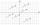Write the parametric equations of height hc in triangle ABC: A = [5; 6], B = [- 2; 4], C = [6; -1]
• Isosceles triangleIn an isosceles triangle ABC with base AB; A [3,4]; B [1,6] and the vertex C lies on the line 5x - 6y - 16 = 0. Calculate the coordinates of vertex C.
• Construct 8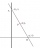Construct an analytical geometry problem where it is asked to find the vertices of a triangle ABC: the vertices of this triangle must be the points A (1,7) B (-5,1) C (5, -11). the said problem should be used the concepts of: distance from a point to a li
• Points in space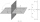There are n points, of which no three lie on one line and no four lies on one plane. How many planes can be guided by these points? How many planes are there if there are five times more than the given points?